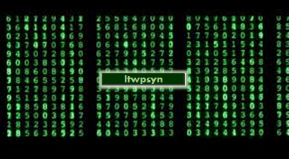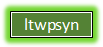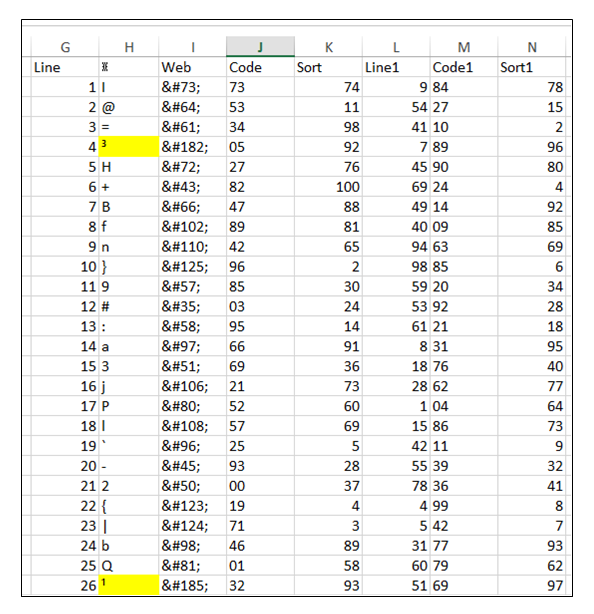1000 Names ltwpsyn® encryptionWelcome to ltwpsyn® encryption

Outline on the ltwpsyn algorithm and its use

The ltwpsyn encryption algorithm works as follows:

Each copy of the Encryption program has a number of allocated sequences, the generic free program uses sequence 1000000000 and sequence 1010001456. Each allocated sequence contains a different sort sequence by date range. The date when an encryption is made plus the allocated sequence used will determine the sort sequence used to make the Cipher used in the Encryption. This is why the date of the encryption is stored in the order YYYYMMDD.The 100 characters allocated a cipher are sorted into the original order Column G. The allocated sequence is obtained from an internet page (there is one page per copy of the program) for the day the encryption is made and loaded into column L. The data is then sorted by column L.

The password which has been entered and which must be greater than 50 characters long is then used to make a cipher for each item in the H column.
The cipher is calculated by taking the ASCII of the character for which a cipher is being made, so if "X" was the first letter then the cipher would be made by taking ASCII for X, which is 88, add the line number which is 1, then going through the password using the ASCII for each character first Multiply the ASCII value of the 1st character, Add the ASCII value of the 2nd, Divide the ASCII value of the 3rd, subtract the ASCII value of the 4th etc for the length of the password.
The cipher is then the right 2 characters of the result, before the cipher is allocated the column M column (Code1) is checked to see if the Cipher has been used and if so then the next number is checked and if available is used.

Each encryption uses a mixing sequence to mix up the 100 characters in each line. There are 200 mixing sequences for each cipher sequence created. A record of the mixing sequences is kept so that the sequence is not used again for 100 encryptions.

The mixing sequence is obtained simply by sorting the Data by the H column, then if the mixing sequence is say 188 then in the N column (Sort1) starting at line 89 and downwards a sequencial count is added until line 101, in line 2 to 87 the sequence would be from 14 to 100. If the mixing sequence was 288 then an upwards sequencial count is added until line 2, and line 101 until line 90 would be from 89 to 100. The data is then sorted by the cipher column M.
The Personal Identification password is used in a similar way to make a cipher in the J column which with the Sort sequence in the K column is used to decrypt the saved password for the To/From identifier.

The mixing sequence displayed in the final encryption uses the sequence 101 to mix the numbers displayed, for decryption the resulting 50 digit number is unmixed, and 25 2 digit numbers are added together and the right 3 characters provide the mixing sequence used for the encryption.

The final encryption:
To make an encryption once the cipher and the mixing sequence are made all that is required is to substitute each character with the cipher, if the character is not in the list of 100 characters then the ASCII for it is used instead. Once 200 numbers are reached the mixing sequence is then used, so for example if the first number in the mixing sequence was 10 then 17 the 10th number becomes the first number in the new line 1, and 110th number becomes the first number in the new line 2 and the 17th number becomes the 2nd number in the new line 2, and the 117th number becomes the 2nd number in the new line 1. This means that each line will only contain 50 characters from the cipher for that line and 50 characters from another line.
When complete the 10th line is the first line and the 17th line the 2nd etc. The process repeats in blocks of 100 lines of 100 characters. Each 100 lines will contain approximately 5,000 characters in a block.

The ltwpsyn encryption algorithm complexity:
With the generic Free program the allocated sequence is known and the difficulty of breaking the encryption lies in the password. An 80 digit password should require 100 factorial (100!) combinations to be solved. The password required of 50 characters equals about 70 factorial combinations making the encryption quite difficult to break.
If the allocated sequence is NOT known the encryption becomes far more difficult to solve. Because the allocated sequence is random and when used with the password changes the cipher result with the same password the number of calculations should be 100 factorial times the number of mix sequences 200.
The ltwpsyn encryption algorithm produces deceptively complex results, the mixing sequences cannot be distinguished and the cipher changes for the generic Free program monthly so is quite dynamic. The allocated sequence for the final release will change daily, and an allocated sequence would only be used by 2 individuals or a group of individuals but the encryption and decryption process is rapid.

The ltwpsyn encryption algorithm going forward:
I have used the algorithm to encrypt the internet and to encrypt Emails. I will be releasing a Free program that encrypts other languages especially simplified and traditional chinese quite soon.
In my White paper I discussed the possibility of making 新浪微博.com and while I will be able to encrypt in 中文 for Excel versions which do support the Unicode and Unichar formula I cannot made a file with the name 新浪微博.com using VBA and Excel. For versions of Excel that do support the Unicode and Unichar formula I will be able to do this, and I will create a demonstration example of this soon!

If you would like to have a encrypted site made for you or be involved in this project then contact me